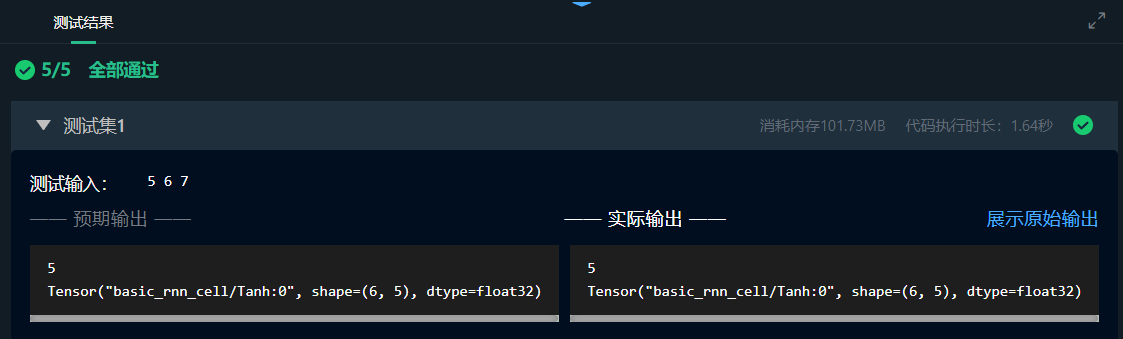# 前言

1. 学习单步的RNN：RNNCell

# 2.相关知识

1. RNNCell 的基本原理和功能；
2. BasicRNNCell 类的常用属性和方法。

## 单个RNNCell的构建

``````cell=tf.nn.rnn_cell.BasicRNNCell(num_units=5)
``````

## RNNCell能做什么

``````cell=tf.nn.rnn_cell.BasicRNNCell(num_units=5)
``````

``````x1=tf.placeholder(tf.float32,[batch_size,n_inputs])
``````

batch_size 代表批量输入值的大小, n_inputs 代表单个输入值的维度

``````cell=tf.nn.rnn_cell.BasicRNNCell(num_units=n_units)
``````

h0=cell.zero_state(batch_size=batch_size,dtype=tf.float32)
output ， h1 分别代表了这个 RNNCell 的输出和当前状态。

``````output,h1=cell.__call__(x1,h0)
``````

``````print(h1)
``````

# 4.笔者答案

``````
import tensorflow as tf

def creatRNNCell(a,b):

cell=tf.nn.rnn_cell.BasicRNNCell(num_units=a)
c=3

x1=tf.placeholder(tf.float32,[b,c])

h0=cell.zero_state(batch_size=b,dtype=tf.float32)

output,h1=cell.__call__(x1,h0)

print(output)

``````

## 通过截图# ; 总结

1. 学习单步的RNN：RNNCell

Original: https://blog.csdn.net/qq_42856609/article/details/122737931
Author: Zhang Wenhao
Title: 1. 学习单步的RNN：RNNCell